># 从 0 开始学习 C 语言：详细分析常见「类型」在内存中的存储

## 1. char 类型内存分析

#include <stdio.h>

int main(void) {
char c = 'A';
printf("c ANSI = %d, c address = 0X%X", c, &c);
// 这里断点调试
getchar();
return 0;
}


c ANSI = 65, c address = 0X15FCFB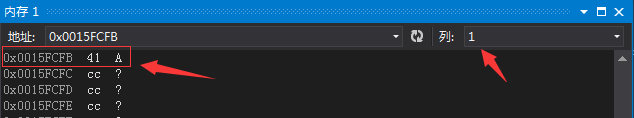0x0015FCFB  41  A

1. 0x0015FCFB 是变量 c 的内存地址
2. 0x41 = 65 = A = 0100 0001 B

## 2. int 类型内存分析

int 占 4 个字节，你理解了上面的例子，举一反三后你应该已经知道了 int 在内存中的存储方式，如果还有点迷糊，继续看这个例子。

#include <stdio.h>

int main(void) {
// 2147483647 = 0x 7F FF FF FF
int i = 2147483647;
printf("i address = 0X%X", &i);
// 这里断点调试
getchar();
return 0;
}


i address = 0X3BFECC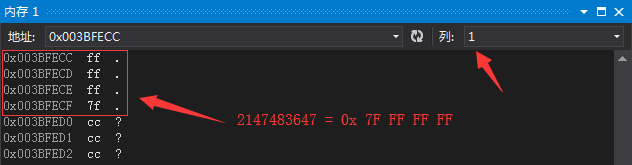0x003BFECC  ff  .
0x003BFECD  ff  .
0x003BFECE  ff  .
0x003BFECF  7f  .


int 是不是很简单，那来个稍微复杂点的，分析下数组吧。

## 3. 数组类型内存分析

#include <stdio.h>

int main(void) {
char c = { 'a', 'b', 'c' };
printf("c address = 0X%X, c address = 0X%X", &c, &c);
// 这里断点调试
getchar();
return 0;
}


c address = 0x3CFB94, c address = 0x3CFB94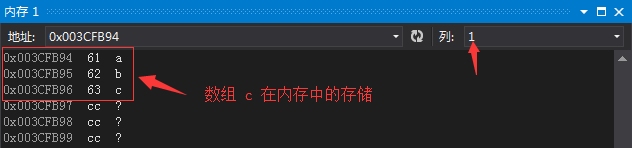0x003CFB94  61  a
0x003CFB95  62  b
0x003CFB96  63  c


## 4. 指针类型内存分析

#include <stdio.h>

int main(void) {
// 2147483647 = 0x 7F FF FF FF
int i = 2147483647;
int *p = &i;
printf("&p = 0X%X, p = &i = 0X%X, sizeof(p) = %d", &p, &i, sizeof(int *));
// 这里断点调试
getchar();
return 0;
}


32 位配置下的运行结果如下：

&p = 0x46F860, p = &i = 0x46F86C, sizeof(p) = 4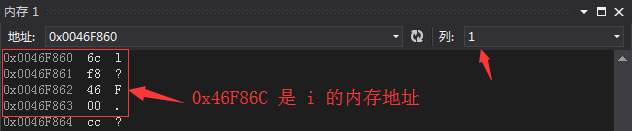## 5. 函数指针内存分析

#include <stdio.h>

// 函数名其实是一个函数指针变量
void fun(void) {
printf("I'm fun.\n");
}

// &fun = fun
int main(void) {
void (*p1)(void) = fun;
printf("&p1 = 0X%X, p1 = fun = 0X%X，&fun = 0X%X\n", &p1, fun, &fun);
p1();

void(*p2)(void) = &fun;
printf("&p1 = 0X%X, p2 = fun = 0X%X，&fun = 0X%X\n", &p2, fun, &fun);
(*p2)();

// 这里断点调试
getchar();
return 0;
}


&p1 = 0X3BF9E0, p1 = fun = 0X13811EF，&fun = 0X13811EF
I'm fun
&p2 = 0X3BF9D4, p2 = fun = 0X13811EF，&fun = 0X13811EF
I'm fun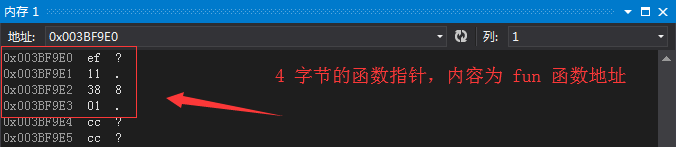## 请一定要实践！

ok，下次见。DLonng at 05/17/18